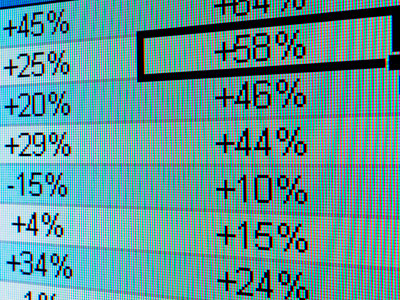# Models 01

This KS3 ICT quiz takes a look at models. Computers can be used for modelling real life situations using mathematical methods. This can be useful in many ways. Spreadsheets can be used to perform many different calculations and are therefore ideal for simple modelling. One everyday use would be to model the finances of your household. The first stage of modelling is to collect and input existing data. Next, you need to decide on how the data is going to be processed and come up with the mathematical expressions to carry out the processing. Finally, you can look at the results. It is then possible to change the input data and see what effect it will have on the results.

When using a spreadsheet, the work space is divided into rectangles called cells. They are arranged in rows and columns and each have a unique reference e.g. cell B3 or cell AB97. Only one cell is active at a time - the cell into which you are entering data. You can enter text, numbers or formulae into a cell but not a combination of them. A formula is a mathematical expression that will carry out a calculation. The calculation can use numbers from different cells.

1.
Spreadsheets are applications designed to .......
do the same job as databases only they are cheaper
give you a large desktop to work on
help accountants
perform calculations on data in a convenient way
You can use your mouse to select a cell on the screen
2.
Typical uses of spreadsheets would not include .......
creating invoices
doing basic calculations on a few numbers
producing charts and graphs
simple databases
Mental arithmetic or using a calculator would be faster
3.
The work space is divided into rectangles called .......
bells
cells
sells
shells
The size of the cell can be adjusted to suit the contents
4.
Each cell may contain .......
numbers and text and formulae at the same time
numbers and text at the same time
numbers only
numbers or text or formulae
Each cell should only contain one type of data at a time
5.
Cells are organised in rows and .......
boxes
columns
layers
shelves
This helps to keep your spreadsheet model organised and easier to follow
6.
A cell is identified by the row and column it is in. They are .......
its identifier
its postcode
the cell location
Cell B2 is found in column B row 2
7.
What do we call a cell being worked on?
Active cell
Calc cell
Current cell
Live cell
You can only type text, symbols and numbers into one cell at a time
8.
A cell can contain a 'formula'. A formula is .......
an instruction telling the spreadsheet what actions to take
an instruction telling the user what actions to take
a chemical equation
None of these
You need to use your knowledge of maths to work out what a formula should be
9.
A formula in a cell usually begins with a .......
#
~
^
=
Note - not all spreadsheets use = this way
10.
A cell can be referred to in a formula using a relative or .......